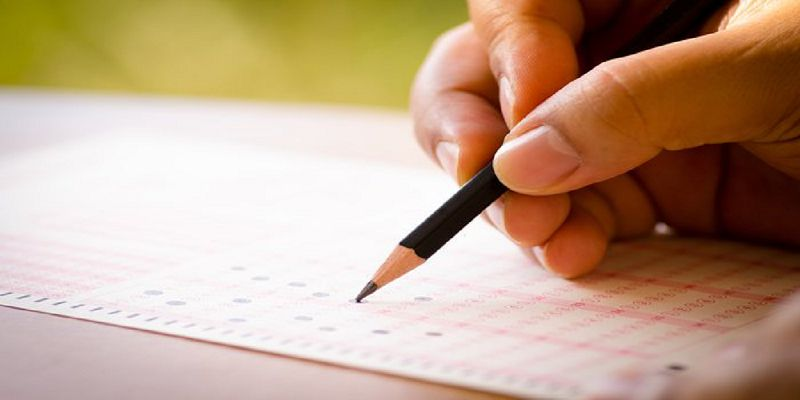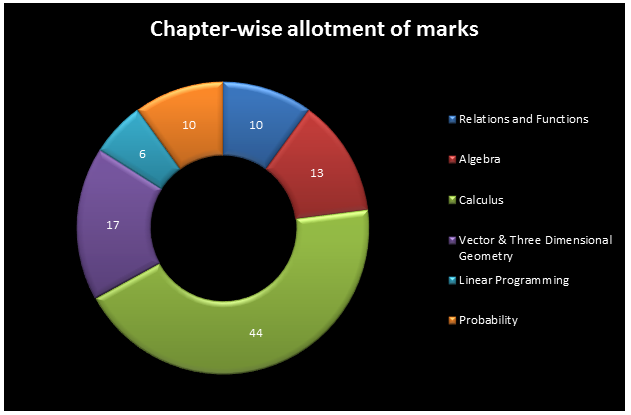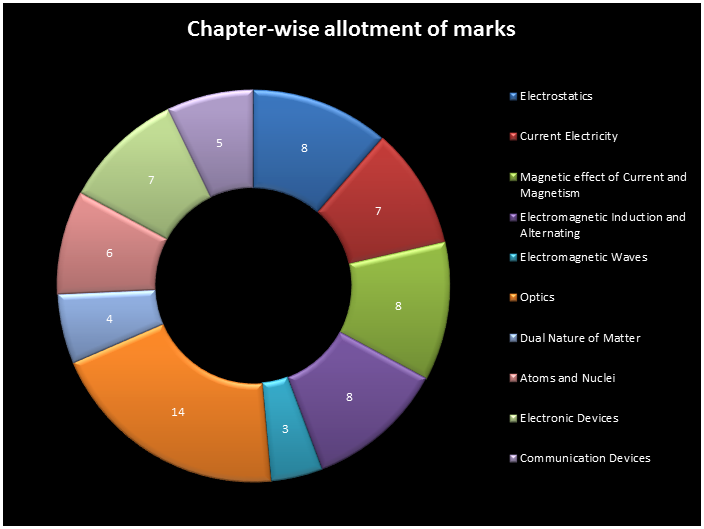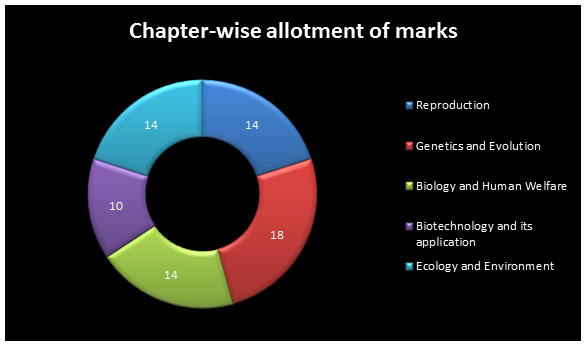Register for Maths, Science, English, Reasoning Olympiad Exams Register here | Book Free Demo for Live Olympiad Classes here | Check Olympiad Exam Dates here | Buy Practice Papers for IMO, IOM, HEO, IOEL etc here | Login here to participate in all India free mock test on every Saturday

# CBSE Class 12th Marking SchemeBefore we start preparing for our board exams it’s necessary that we first look at the marking scheme as it is the guide to allocating marks to the candidates. Given below is a brief of the marking scheme which shows the weight age given to each section for the Science students.

## Mathematics Marking Scheme:

The marking scheme for mathematics is very important as it is a subject where we can score comparatively better with just a little practice. The maximum weight age in this subject is given to the Calculus section least to the linear equations. The duration is 3 hours with maximum marks 100.## Physics Marking Scheme:

The Physics paper is divided into two sections – Theory and Practical. The theory part carries 70 marks and the practical part carries 30 marks. The topic of Optics carries the maximum weight age and the duration here also is 3 hours for the theory exam.## Chemistry Marking Scheme:

The chemistry marking scheme is quite similar to that of physics. Theory carries 70 marks and practical carries 30 marks. Almost all the sections have the same weight age.## Biology Marking Scheme:

Here also 70 marks are for theory and 30 marks are for practical. The topic if Genetics and evolution carries the maximum weight age.For the subjects- Physics, Chemistry and Biology, the marking scheme for the practical part is as follows:

• Two experiments from different sections – (8+8) 16 marks
• Practical record (experiments and activities) – 6 marks
• Project work – 3 marks
• Viva (experiment and project) – 5 marks

Students should not only concentrate on sections with more weight age but devote equal time to each and every section so that they score good. For that they should make a proper study plan and time table.